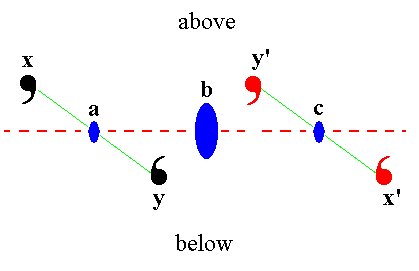```
```
Remark on implied rotational symmetry
```

```

back to homepage

back to main document

(These links are only appropriate for readers who arrived directly on this particular page, i.e. not via the Homepage.)

```
```
Concerning the Figures of stereograms we often spoke about motifs being generated, forming a certain pattern, etc. However, in fact, not motifs are depicted there, but projections of face poles. But because they represent motifs (i.e. real entities in crystals) we also call them motifs, where appropriate.

At (8) in Figure 11a (main document) we stated that "the resulting motif pattern turns out to be consistent with six-fold rotational symmetry of the n-fold axis (i.e. the axis perpendicular to the plane of the drawing)."
Of course this is not evident just like that, but the next Figures will show that indeed the motif pair -- in stereograms represented by the projections of face poles and then depicted as small solid circles, standing for a projection from above, or small open circles, standing for a projection from below the projection plane -- is horizontally repeated about the vertical axis.Figure 11aa. Part of a stereogram (see (3) in Figure 11a in the main document) showing two pairs of (projections of) face poles (sometimes called motifs) related by a 2-fold rotation axis. Also the members of each pair are related to each other by a 2-fold rotation axis. The next Figure shows that these pairs are repetitions of each other, i.e. they are congruent.

```
```Figure 11aaa. This Figure depicts the same situation as the above Figure, but now seen in a direction perpendicular to that of the latter. The (projections of the) face poles are now depicted by what they represent :   motifs (drawn as comma's).
We see the three 2-fold rotation axes a, b and c (See Figure 11aa), depicted as blue solid ellipses. The axis b, relating the two motif pairs is emphasized. The dashed red line represents the projection plane (here seen, not as a plane but as a line, in virtue of our direction of view).
The 2-fold rotation axis b maps x onto x', and y onto y'.
We can clearly see that the two pairs are congruent.
In virtue of the multiplication of 2-fold axes, as described in Figure 11a (main document), these motif pairs will also be multiplied, resulting in a total of six congruent motif pairs, consistent with a 6-fold vertical rotation axis.

****************

back to homepage

back to main document

(These links are only appropriate for readers who arrived directly on this particular page, i.e. not via the Homepage.)

```
```